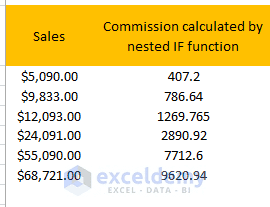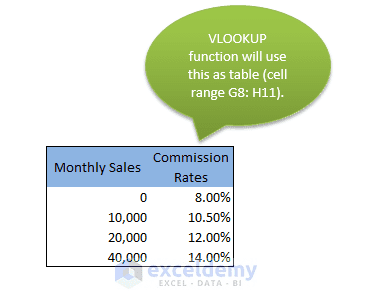Disclosure: This post may contain affiliate links, meaning when you click the links and make a purchase, we receive a commission.

# How to Use Excel VBA User Defined Function in Formula

Excel VBA provides two procedures. One is functions also known as the user-defined functions another one is subroutines. In this tutorial, I am going to discuss how to use the Excel VBA user-defined function in Excel formula.

The Excel VBA user-defined function is a process that is written in VBA which accepts some inputs &  in return gives a result when the function is called.

## Example of an Excel VBA User-Defined Function

Before entering into details, at first, I introduce you to the method Speak(). This method has the following syntax:

expression.Speak (Text, [SpeakAsync], [SpeakXML], [Purge])

The expression represents a Speech object.

Though Speak() method can take three arguments, but Text argument is the required one, the rest are optional. I shall use Speak() function by passing only Text argument. So, in this case, it is a function of one argument. This method uses Excel text-to-speech generator to “speak” the text passed to it through Text argument.

```Function SpeakTheText(text)
Application.Speech.Speak (text)
End Function
```

Note: To hear the voice, your system must be set up to play sound.

## Using VBA function in excel cell

For example, if you want to use the SpeakTheText() function to hear the content of cell A1, you have to use the following formula:

`=SpeakTheText(A1)`

You can use this function in a slightly more complex formula, as shown below. In this example, the argument is a text string (“Good achievement”) rather than a cell reference.

`=IF(SUM(A:A)&amp;gt;1000, SpeakTheText("Goal achievement"),)`

The above formula calculates the sum of the values in Column A. When that sum exceeds 1,000, you will hear “Good achievement”.

When you use the SpeakTheText function in a worksheet formula, the function always returns 0, because this function is returning nothing. In another word, I can say, there is no value assigned to the function’s name (SpeakTheText) variable.

Read More: An Excel VBA function with a range argument

## Another Example of Excel VBA User-Defined Function

Okay, now I’m going to deal with a more complex function. This function is designed for a sales manager who calculates monthly commission that he has to pay to his salespersons. To boost up the sales of the company, the commission rate structure is based on the amount sold— those who will sell more will earn a higher commission rate.

The function takes the sales amount through the only argument (Sales) and returns the commission earned by a salesperson. The function will calculate the commission according to the following table:

### Table: Monthly Sales Target & Commission Rates

Monthly SalesCommission Rate
0-\$9,9998.0%
\$10,000-\$19,99910.5%
\$20,000-\$39,99912.0%
\$40,000+14.0%

You can use more than one method to calculate commissions for various sales amounts.

### Using Nested IF function

Assume that you have the sales amount for a particular salesperson in a worksheet cell A4. Now you can use the following formula:

```=
IF (AND (A4>=0, A4<=9999.99), A4*0.08,
IF (AND (A4>=10000, A4<=19999.99), A4*0.105,
IF (AND (A4>=20000, A4<=39999.99), A4*0.12,
IF (A4>=40000, A4*0.14, 0))))
```Commission calculated by if function.

This approach isn’t a good solution for the following reasons:

• Firstly, the formula is undoubtedly complex and not easy to understand.
• Secondly, the commission rates are hard-coded into the formula. If the commission structure changes in future, it would be tough and toilsome to modify the formula.

### Using VLOOKUP function

A simple and better solution is using a VLOOKUP table. I have entered the data of the above table (Monthly Sales Target & Commission Rates) in cell range G8: H11. Click this link to know about VLOOKUP function, if you don’t know.VLOOKUP table for the data that calculates the commission rates for sales target.

Note: It is important to note that I have used 0 for the data range 0-9999, 10,000 for 10,000-19,999, 20,0000 for 20,000-39,999 and 40,000 for 40,000+. When I do so, Excel searches data < 10,000 in 0 row, 10,000 ≤ data ≤ 19,999 in 10,000 row, 20,000 ≤ data ≤ 39,999 in row 20,000 and data ≥ 40,000 in row 40,000.

`=VLOOKUP(A4, \$G\$8: \$H\$11, 2)*A4`

Use this function in other cells to calculate the sales commission earned by the sales force.

## Using VBA Function in Excel Formula

Like the following one, you can create a custom function to calculate the commission earning by the sales force.

```Function CommissionEarning(Sales)
'Calculates sales commissions
Rate1 = 0.08
Rate2 = 0.105
Rate3 = 0.12
Rate4 = 0.14
Select Case Sales
Case 0 To 9999.99
CommissionEarning = Sales * Rate1
Case 10000 To 19999.99
CommissionEarning = Sales * Rate2
Case 20000 To 39999.99
CommissionEarning = Sales * Rate3
Case Is &amp;gt;= 40000
CommissionEarning = Sales * Rate4
End Select
End Function
```

I have used the custom function in cell D4 using the argument A4, as A4 holds the sales. Then I have copied this function for other cells. The figure below shows this: